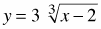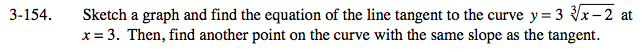### Home > CALC > Chapter Ch3 > Lesson 3.4.1 > Problem3-154

3-154.

Sketch a graph and find the equation of the line tangent to the curveat x = 3. Then, find another point on the curve with the same slope as the tangent. Homework Help ✎equation of tangent at x = 3:
yy1 = m(xx1)

equation of tangent at x = 3:
y − 3 = 1(x − 3)
y = x − 3 + 3
y = x

To find another point with the same slope, consider the symmetry of the curve.

m = y '(3)

$\text{since }y= 3\sqrt{x-2}=3(x-2)^{\frac{1}{3}}$

$\text{so }y'= (x-2)^{-\frac{2}{3}}= \frac{1}{(x-2)^{\frac{2}{3}}}$

and y '(3) = 1 = m

x1 = 3

$y_{1} = 3\sqrt{(3)-2}=3$

Use the eTool below to explore the graph of the curve.
Click the link at right for the full version of the eTool: Calc 3-154 HW eTool